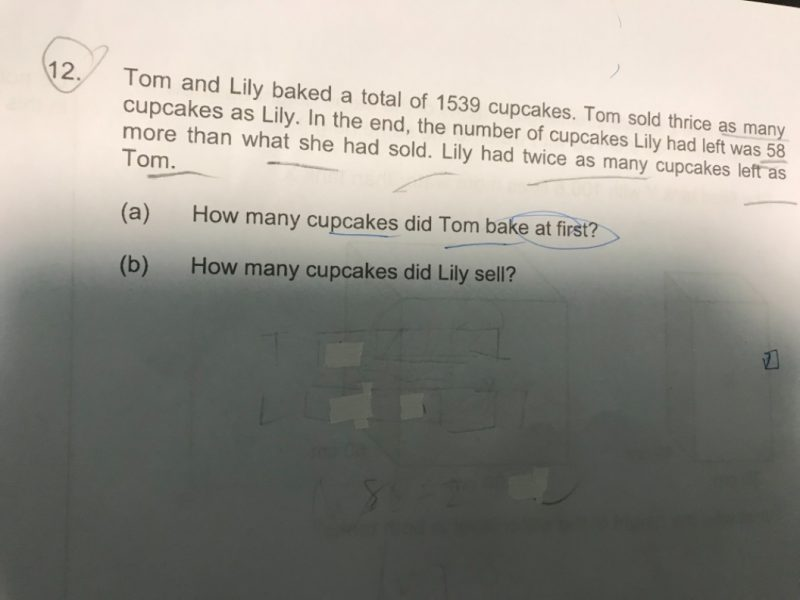# QuestionHi ,

Can anyone help to solve this question ?

Thanks

Given

i. Tom sells 3 times than Lily

ii. Lily has 58 more cupcakes left than what she sold.

iii. Tom has half the cupcakes as Lily

With the given info, we form the table below.

 Sold Left Total baked Tom 6U 1U + 29 7U + 29 Lily 2U 2U + 58 4u + 58

Total cup cakes = 1539.

7U + 29 + 4U + 58 = 1539.

11U + 29 + 58 = 1539

11U = 1452, 1U = 132

(a) Total cupcakes Tom baked = 7u + 29 =  924 + 29  = 953

(b) Lily sold = 2U = 132 x 2 = 264

0 Replies 0 Likes